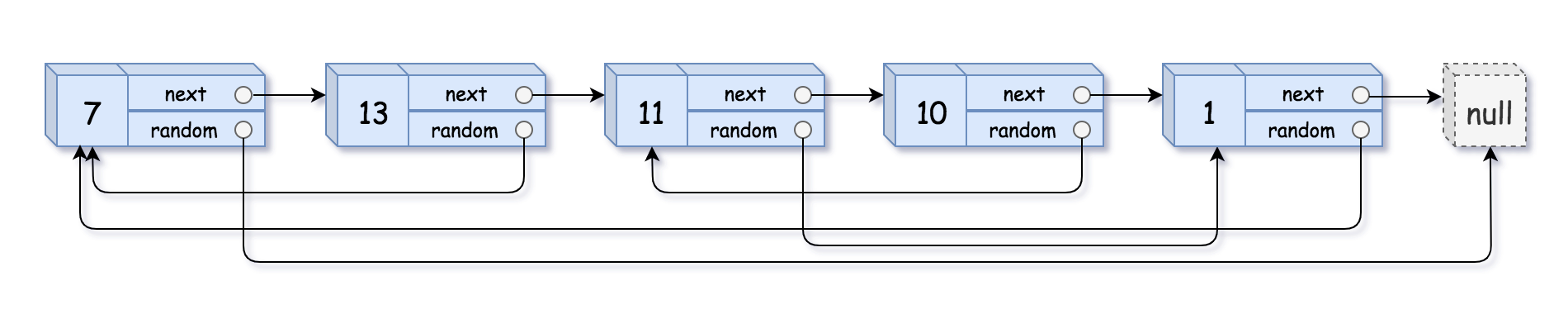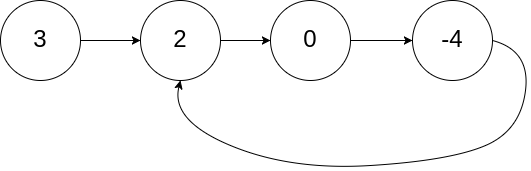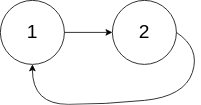# leetcode 148. Sort List

## 题目描述

Sort a linked list in O ( n log n ) time using constant space
complexity.

Example 1:

``````Input: 4->2->1->3
Output: 1->2->3->4``````

Example 2:

``````Input: -1->5->3->4->0
Output: -1->0->3->4->5``````

Tags: Linked List, Sort

Difficulty: Medium



# leetcode 138. Copy List with Random Pointer

## 题目描述

A linked list is given such that each node contains an additional random
pointer which could point to any node in the list or null.

Return a deep
copy
of the list.

The Linked List is represented in the input/output as a list of `n` nodes.
Each node is represented as a pair of `[val, random_index]` where:

• `val`: an integer representing `Node.val`
• `random_index`: the index of the node (range from `0` to `n-1`) where random pointer points to, or `null` if it does not point to any node.

Example 1:Input: head = [[7,null],[13,0],[11,4],[10,2],[1,0]] Output: [[7,null],[13,0],[11,4],[10,2],[1,0]]

Example 2:Input: head = [[1,1],[2,1]] Output: [[1,1],[2,1]]

Example 3:Input: head = [[3,null],[3,0],[3,null]] Output: [[3,null],[3,0],[3,null]]

Example 4:
Input: head = [] Output: [] Explanation: Given linked list is empty (null pointer), so return null.

Constraints:

• `-10000 <= Node.val <= 10000`
• `Node.random` is null or pointing to a node in the linked list.
• Number of Nodes will not exceed 1000.

Tags: Hash Table, Linked List

Difficulty: Medium



# leetcode 25. Reverse Nodes in k-Group

## 题目描述

Given a linked list, reverse the nodes of a linked list k at a time and
return its modified list.

k is a positive integer and is less than or equal to the length of the
linked list. If the number of nodes is not a multiple of k then left-out
nodes in the end should remain as it is.

Example:

Given this linked list: `1->2->3->4->5`

For k = 2, you should return: `2->1->4->3->5`

For k = 3, you should return: `3->2->1->4->5`

Note:

• Only constant extra memory is allowed.
• You may not alter the values in the list’s nodes, only nodes itself may be changed.

Tags: Linked List

Difficulty: Hard



# leetcode 328. Odd Even Linked List

## 题目描述

Given a singly linked list, group all odd nodes together followed by the even
nodes. Please note here we are talking about the node number and not the value
in the nodes.

You should try to do it in place. The program should run in O(1) space
complexity and O(nodes) time complexity.

Example 1:

``````Input:1->2->3->4->5->NULL
Output:1->3->5->2->4->NULL``````

Example 2:

``````Input: 2->1->3->5->6->4->7->NULL
Output:2->3->6->7->1->5->4->NULL``````

Note:

• The relative order inside both the even and odd groups should remain as it was in the input.
• The first node is considered odd, the second node even and so on …

Tags: Linked List

Difficulty: Medium

# leetcode 142. Linked List Cycle II

## 题目描述

Given a linked list, return the node where the cycle begins. If there is no
cycle, return `null`.

To represent a cycle in the given linked list, we use an integer `pos` which
represents the position (0-indexed) in the linked list where tail connects to.
If `pos` is `-1`, then there is no cycle in the linked list.

Note: Do not modify the linked list.

Example 1:
Input: head = [3,2,0,-4], pos = 1 Output: tail connects to node index 1 Explanation: There is a cycle in the linked list, where tail connects to the second node.Example 2:
Input: head = [1,2], pos = 0 Output: tail connects to node index 0 Explanation: There is a cycle in the linked list, where tail connects to the first node.Example 3:
Input: head = , pos = -1 Output: no cycle Explanation: There is no cycle in the linked list.Follow-up :
Can you solve it without using extra space?

Tags: Linked List, Two Pointers

Difficulty: Medium

# leetcode 141. Linked List Cycle

## 题目描述

Given a linked list, determine if it has a cycle in it.

To represent a cycle in the given linked list, we use an integer `pos` which
represents the position (0-indexed) in the linked list where tail connects to.
If `pos` is `-1`, then there is no cycle in the linked list.

Example 1:

``````Input: head = [3,2,0,-4], pos = 1
Output: true
Explanation: There is a cycle in the linked list, where tail connects to the second node.``````Example 2:

``````Input: head = [1,2], pos = 0
Output: true
Explanation: There is a cycle in the linked list, where tail connects to the first node.``````Example 3:

``````Input: head = , pos = -1
Output: false
Explanation: There is no cycle in the linked list.``````Follow up:

Can you solve it using O(1) (i.e. constant) memory?

Tags: Linked List, Two Pointers

Difficulty: Easy

# leetcode 237. Delete Node in a Linked List

## 题目描述

Write a function to delete a node (except the tail) in a singly linked list,
given only access to that node.

Given linked list – head = [4,5,1,9], which looks like following:Example 1:

``````Input: head = [4,5,1,9], node = 5
Output: [4,1,9]
Explanation: You are given the second node with value 5, the linked list should become 4 -> 1 -> 9 after calling your function.``````

Example 2:

``````Input: head = [4,5,1,9], node = 1
Output: [4,5,9]
Explanation: You are given the third node with value 1, the linked list should become 4 -> 5 -> 9 after calling your function.``````

Note:

• The linked list will have at least two elements.
• All of the nodes’ values will be unique.
• The given node will not be the tail and it will always be a valid node of the linked list.
• Do not return anything from your function.

Tags: Linked List

Difficulty: Easy

# leetcode 92. Reverse Linked List II

## 题目描述

Reverse a linked list from position m to n. Do it in one-pass.

*Note: *1 ≤ mn ≤ length of list.

Example:

``````Input: 1->2->3->4->5->NULL, _m_ = 2, _n_ = 4
Output: 1->4->3->2->5->NULL``````

Tags: Linked List

Difficulty: Medium

# leetcode 82. Remove Duplicates from Sorted List II

## 题目描述

Given a sorted linked list, delete all nodes that have duplicate numbers,
leaving only distinct numbers from the original list.

Example 1:

``````Input: 1->2->3->3->4->4->5
Output: 1->2->5``````

Example 2:

``````Input: 1->1->1->2->3
Output: 2->3``````

Tags: Linked List

Difficulty: Medium

# leetcode 61. Rotate List

## 题目描述

Given a linked list, rotate the list to the right by k places, where k is
non-negative.

Example 1:

``````Input: 1->2->3->4->5->NULL, k = 2
Output: 4->5->1->2->3->NULL
Explanation:
rotate 1 steps to the right: 5->1->2->3->4->NULL
rotate 2 steps to the right: 4->5->1->2->3->NULL``````

Example 2:

``````Input: 0->1->2->NULL, k = 4
Output: 2->0->1->NULL
Explanation:
rotate 1 steps to the right: 2->0->1->NULL
rotate 2 steps to the right: 1->2->0->NULL
rotate 3 steps to the right: 0->1->2->NULL
rotate 4 steps to the right: 2->0->1->NULL``````

Tags: Linked List, Two Pointers

Difficulty: Medium

:D 一言句子获取中...